# True or False (Problems 1 through 10) 1. The change in entropy of a closed system...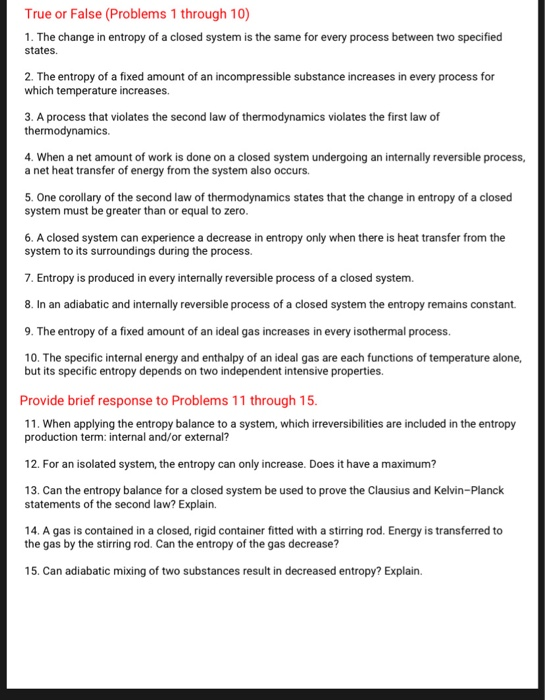True or False (Problems 1 through 10) 1. The change in entropy of a closed system is the same for every process between two specified states 2. The entropy of a fixed amount of an incompressible substance increases in every process for which temperature increases 3. A process that violates the second law of thermodynamics violates the first law of thermodynamics. 4. When a net amount of work is done on a closed system undergoing an internally reversible process, a net heat transfer of energy from the system also occurs. 5. One corollary of the second law of thermodynamics states that the change in entropy of a closed system must be greater than or equal to zero. 6.A closed system can experience a decrease in entropy only when there is heat transfer from the system to its surroundings during the process 7. Entropy is produced in every internally reversible process of a closed system. 8. In an adiabatic and internally reversible process of a closed system the entropy remains constant 9. The entropy of a fixed amount of an ideal gas increases in every isothermal process. 10. The specific internal energy and enthalpy of an ideal gas are each functions of temperature alone, but its specific entropy depends on two independent intensive properties. Provide brief response to Problems 11 through 15 11. When applying the entropy balance to a system, which irreversibilities are included in the entropy production term: internal and/or external? 12. For an isolated system, the entropy can only increase. Does it have a maximum? 13. Can the entropy balance for a closed system be used to prove the Clausius and Kelvin-Planck statements of the second law? Explain. 14. A gas is contained in a closed, rigid container fitted with a stirring rod. Energy is transferred to the gas by the stirring rod. Can the entropy of the gas decrease? 15. Can adiabatic mixing of two substances result in decreased entropy? Explain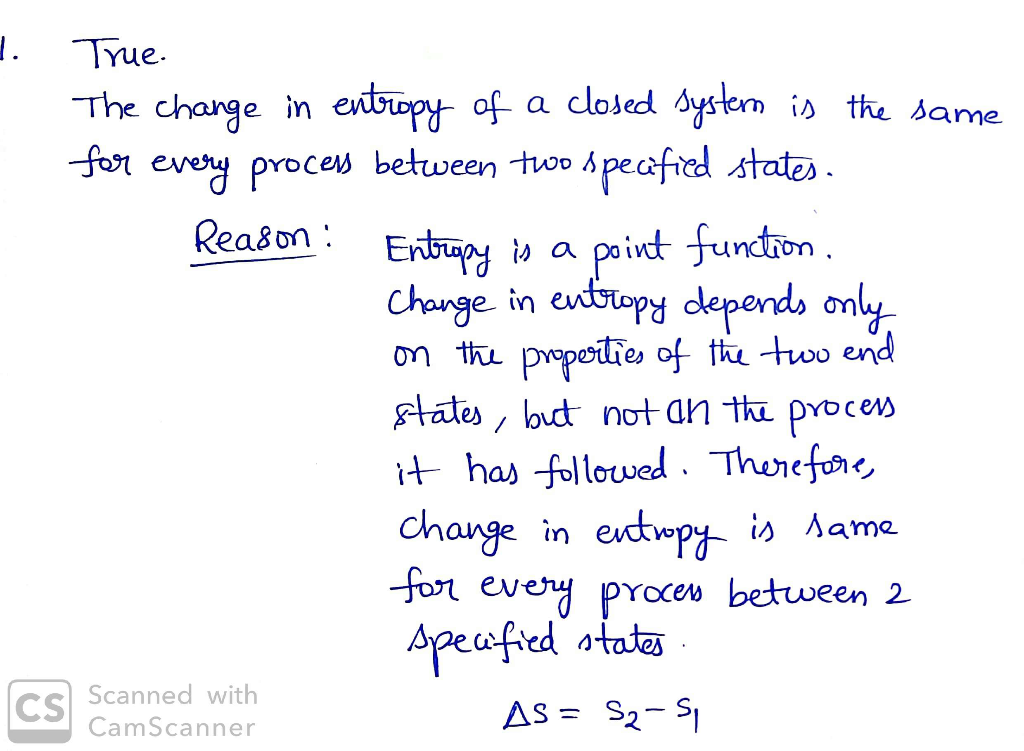#### Earn Coin

Coins can be redeemed for fabulous gifts.

Similar Homework Help Questions
• ### 5. (15) A closed system undergoes a process where heat transfer is allowed along the system...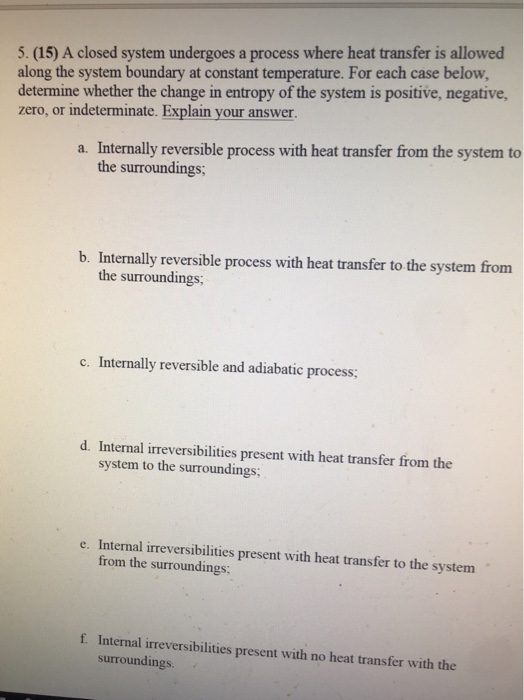5. (15) A closed system undergoes a process where heat transfer is allowed along the system boundary at constant temperature. For each case below, determine whether the change in entropy of the system is positive, negative, zero, or indeterminate. Explain your answer. a. Internally reversible process with heat transfer from the system to the surroundings b. Internally reversible process with heat transfer to the system from the surroundings; Internally reversible and adiabatic process; c. d. Internal irreversibilities present with heat...

• ### The statements in the following list all refer to thermodynamics and entropy. Check the boxes of...

The statements in the following list all refer to thermodynamics and entropy. Check the boxes of the THREE CORRECT statements. 1. Entropy is similar to energy and obeys a conservation law. 2. In an adiabatic process the change in the internal energy of the gas is the same as the work done on the gas. 3. An ideal gas undergoes a reversible process whereby the pressure of the gas decreases by a factor of 3 but its volume increases by...

• ### c) A closed system undergoes a process for which the system entropy is constant (S2 S)...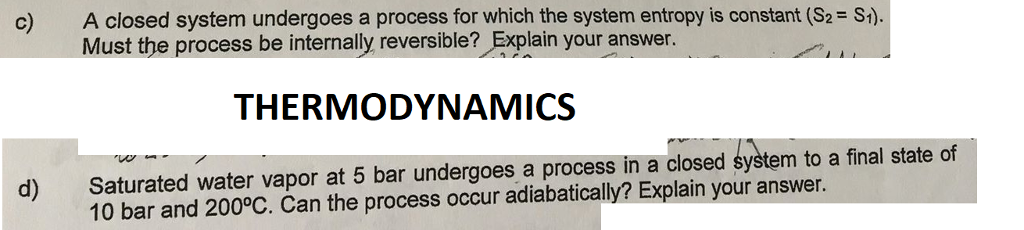c) A closed system undergoes a process for which the system entropy is constant (S2 S) Must the process be internally reversible? Explain your answer. THERMODYNAMICS d) Saturated water vapor at 5 bar undergoes a process in a closed system to a final state of 10 bar and 200°C. Can the process occur adiabatically? Explain your answer.

• ### Please help me solve this urgently ( chemical engineering thermodynamics) A. True or False; answ er...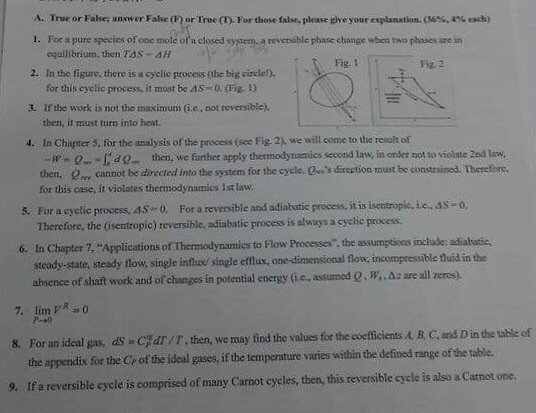Please help me solve this urgently ( chemical engineering thermodynamics) A. True or False; answ er False() orTrue(T). For those false, please give your explanation. (6%,4% each) 1. For a pure species of one mole of'a closed system, a reversible phase change wben two phases are in equilibrium, then TSS-ΔΗ Fig. 1 Fiz 2 2. In the figure, there is a cyclic process (the big circle!). for this cyclic process, it must be AS-O. (Fig D 3. If the work...

• ### Please answer all questions, I will upvote thanks. QUESTION 1 Which of the following statements about...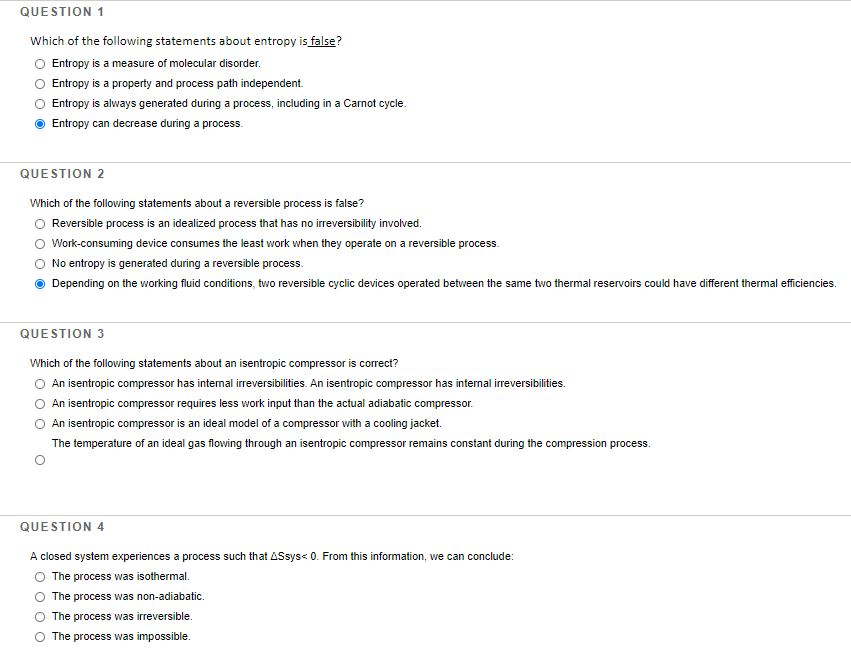Please answer all questions, I will upvote thanks. QUESTION 1 Which of the following statements about entropy is false? Entropy is a measure of molecular disorder. Entropy is a property and process path independent. Entropy is always generated during a process, including in a Carnot cycle. Entropy can decrease during a process. QUESTION 2 Which of the following statements about a reversible process is false? Reversible process is an idealized process that has no irreversibility involved. Work-consuming device consumes the...

• ### The second law of thermodynamics states that the entropy of a closed system always increases. Cellular...

The second law of thermodynamics states that the entropy of a closed system always increases. Cellular systems take disordered substrates to construct highly ordered molecules and organelles. How can photosynthesis be used to make glucose without violating this law? Note: glucose is more a ordered molecule then the CO2 used to construct it.

• ### Q1: The difference between a nonflow (closed) and a filow (open) system is that Nonflow system allows mass transfer while flow system does not Nonflow system allows Flow system Flow energy transfer w...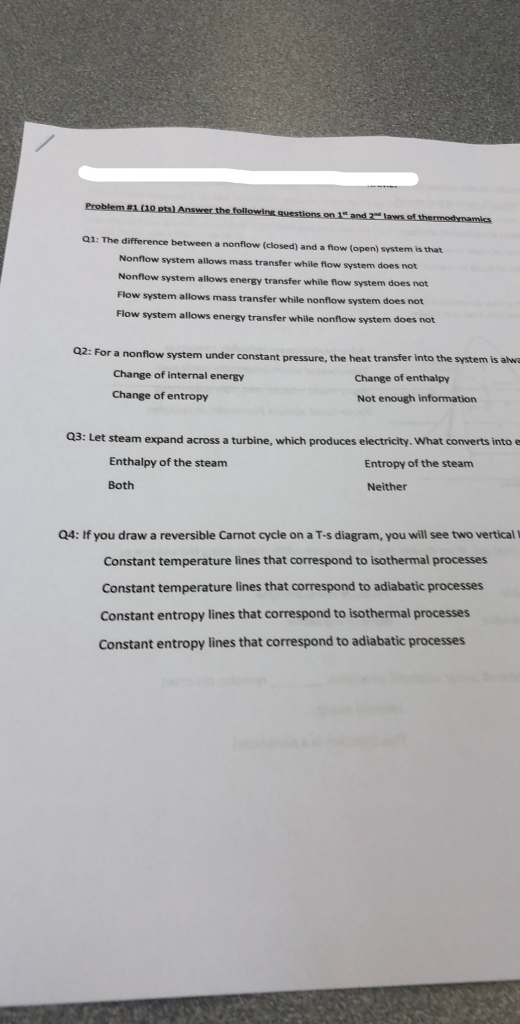Q1: The difference between a nonflow (closed) and a filow (open) system is that Nonflow system allows mass transfer while flow system does not Nonflow system allows Flow system Flow energy transfer while flow system does not allows mass transfer while nonflow system does not system allows energy transfer while nonflow system does not Q2: For a nonflow system under constant pressure, the heat transfer into the system is ahwa Change of internal energy Change of entropy Change of enthalp...

• ### Problem 1. 1 kg of air (assumed ideal gas) contained in a piston-cylinder assembly. The a...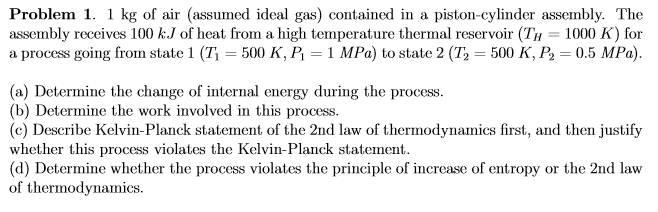Problem 1. 1 kg of air (assumed ideal gas) contained in a piston-cylinder assembly. The a process going from state 1 (T = 500 K, P = 1 MPa) to state 2 (T) = 500 K, P = 0.5 MPa). (a) Determine the change of internal energy during the process. (b) Determine the work involved in this process. (c) Describe Kelvin-Planck statement of the 2nd law of thermodynamics first, and then justify whether this process violates the Kelvin-Planck statement. (d)...

• ### (1) A process has been proposed whereby an ideal gas is taken from P=10 bar and...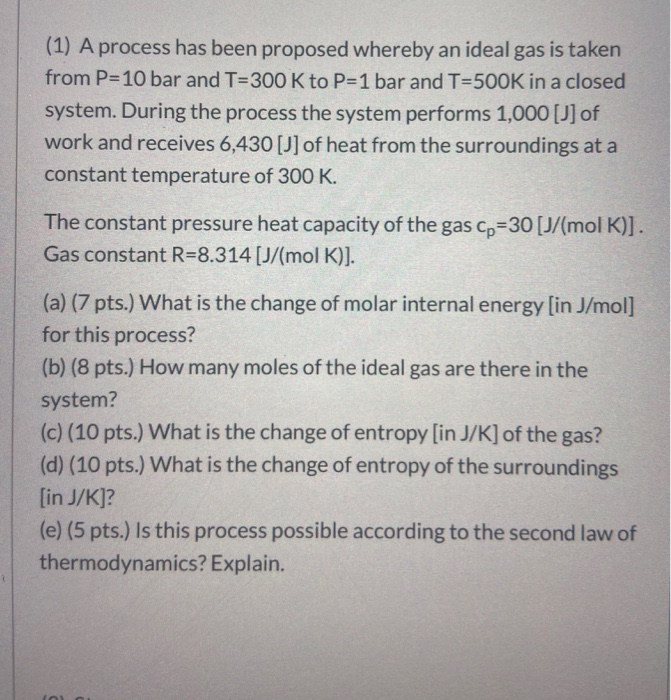(1) A process has been proposed whereby an ideal gas is taken from P=10 bar and T=300 K to P=1 bar and T=500K in a closed system. During the process the system performs 1,000 [J] of work and receives 6,430 [j] of heat from the surroundings at a constant temperature of 300 K. The constant pressure heat capacity of the gas Cp=30 [J/(mol K)]. Gas constant R=8.314 [J/(mol K)]. (a) (7 pts.) What is the change of molar internal energy...

• ### (1) A process has been proposed whereby an ideal gas is taken from P=10 bar and...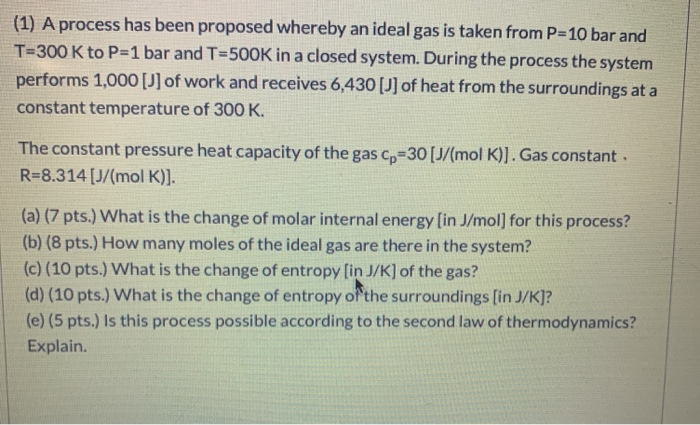(1) A process has been proposed whereby an ideal gas is taken from P=10 bar and T=300 K to P=1 bar and T=500K in a closed system. During the process the system performs 1,000 [j] of work and receives 6,430 [J] of heat from the surroundings at a constant temperature of 300 K. The constant pressure heat capacity of the gas cp=30 [J/mol K)]. Gas constant. R=8.314 [J/(mol K)]. (a) (7 pts.) What is the change of molar internal energy...